# The SIM2D Procedure

### SIMULATE Statement

SIMULATE simulate-options ;

The SIMULATE statement specifies details on the simulation and the covariance model used in the simulation. You can specify the following simulate-options with a SIMULATE statement, which can be abbreviated by SIM.

Table 85.3 summarizes the options available in the SIMULATE statement.

Table 85.3: SIMULATE Statement Options

Option

Description

Simulate-Options

Specifies the number of realizations to produce

Specifies the conditioning variable

Covariance Model Specification

Specifies the angle of the major axis

Specifies the functional form

Specifies the input data set containing parameter values

Specifies the nugget effect for the model

Specifies the range parameter

Specifies the ratio of the minor axis to the major axis

Specifies the scale (or sill) parameter

Specifies the seed to use for the random number generator

Gives the singularity criterion

Specifies the smoothness parameter

Uses information from an input item store for prediction

NUMREAL=number
NUMR=number
NR=number

specifies the number of realizations to produce for the spatial process specified by the covariance model. As a result, the number of observations in the OUTSIM= data set contributed by a given SIMULATE statement is the product of the NUMREAL= value and the number of grid points. This can cause the OUTSIM= data set to become large even for moderate values of the NUMREAL= option.

VAR=(variable-name)

specifies the single numeric variable used as the conditioning variable in the simulation. In other words, the simulation is conditional on the values of the VAR= variable found in the DATA= data set. If you omit the VAR= option or if all observations of the VAR= variable are missing values, then the simulation is unconditional. Since multiple SIMULATE statements are allowed, you can perform both unconditional and conditional simulations with a single PROC SIM2D statement.

#### Covariance Model Specification

You can specify a semivariogram or covariance model in three ways:

• You specify the required parameters SCALE, RANGE, FORM, and SMOOTH (if you specify the MATERN form), and possibly the optional parameters NUGGET, ANGLE, and RATIO, explicitly in the SIMULATE statement.

• You specify an MDATA= data set. This data set contains variables that correspond to the required parameters SCALE, RANGE, FORM, and SMOOTH (if you specify the MATERN form), and, optionally, variables for the NUGGET, ANGLE, and RATIO parameters.

• You can specify an input item store in the RESTORE statement. The item store contains one or more correlation models for one or more direction angles. You can specify these models in the STORESELECT option of the SIMULATE statement to run a simulation.

The three methods are mutually exclusive: you specify all parameters explicitly, they are all are read from the MDATA= data set, or you select a model and its parameters from an input item store. The following simulate-options are related to model specification:

ANGLE=angle
ANGLE=(angle1, …, anglek)

specifies the angle of the major axis for anisotropic models, measured in degrees clockwise from the N–S axis. The default is ANGLE=0.

In the case of a nested semivariogram model with k nestings, you have the following two ways to specify the anisotropy major axis: you can specify only one angle which is then applied to all nested forms, or you can specify one angle for each of the k nestings.

Note: The syntax makes it possible to specify different angles for different forms of the nested model, but this practice is rarely used.

FORM=form
FORM=(form1, …, formk)

specifies the functional form (type) of the semivariogram model. Use the syntax with the single form to specify a non-nested model. Use the syntax with forms formi,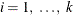, to specify a nested model with k structures. Each of the forms can be any of the following:

CUBIC | EXPONENTIAL | GAUSSIAN | MATERN |
PENTASPHERICAL | SINEHOLEEFFECT | SPHERICAL
CUB | EXP | GAU | MAT | PEN | SHE | SPH

specify the functional form (type).

For example, the syntax

FORM=GAU


specifies a model with a single Gaussian structure. Also, the syntax

FORM=(EXP,SHE,MAT)


specifies a nested model with an exponential, a sine hole effect, and a Matérn structure. Finally

FORM=(EXP,EXP)


specifies a nested model with two structures both of which are exponential.

Note: In the documentation, models are named either by using their full names or by using the first three letters of their structures. Also, the names of different structures in a nested model are separated by a hyphen (-). According to this convention, the previous examples illustrate how to specify a GAU, an EXP-SHE-MAT, and an EXP-EXP model, respectively, with the FORM= option.

All the supported model forms have two parameters specified by the SCALE= and RANGE= options, except for the MATERN model which has a third parameter specified by the SMOOTH= option. A FORM= value is required, unless you specify the MDATA= option or the STORESELECT option.

Computation of the MATERN covariance is numerically demanding. As a result, simulations that use Matérn covariance structures can be time-consuming.

MDATA=SAS-data-set

specifies the input data set that contains parameter values for the covariance or semivariogram model. The MDATA= option cannot be combined with any of the FORM= or STORESELECT options.

The MDATA= data set must contain variables named SCALE, RANGE, and FORM, and it can optionally contain variables NUGGET, ANGLE, and RATIO. If you specify the MATERN form, then you must also include a variable named SMOOTH in the MDATA= data set.

The FORM variable must be a character variable, and it can assume only the values allowed in the explicit FORM= syntax described previously. The RANGE, SCALE and SMOOTH variables must be numeric. The optional variables ANGLE, RATIO, and NUGGET must also be numeric if present.

The number of observations present in the MDATA= data set corresponds to the level of nesting of the covariance or semivariogram model. For example, to specify a non-nested model that uses a spherical covariance, an MDATA= data set might contain the following statements:

data md1;
input scale range form $; datalines; 25 10 SPH ;  The PROC SIM2D statement to use the MDATA= specification is of the form shown in the following: proc sim2d data=...; sim var=.... mdata=md1; run;  This is equivalent to the following explicit specification of the covariance model parameters: proc sim2d data=...; sim var=.... scale=25 range=10 form=sph; run;  The following MDATA= data set is an example of an anisotropic nested model: data md2; input scale range form$ nugget angle ratio smooth;
datalines;
20 8 SPH  5 35 .7   .
12 3 MAT  5 0  .8 2.8
4  1 GAU  5 45 .5   .
;

proc sim2d data=...;
sim var=.... mdata=md2;
run;


This is equivalent to the following explicit specification of the covariance model parameters:

proc sim2d data=...;
sim var=.... scale=(20,12,4) range=(8,3,1) form=(SPH,MAT,GAU)
angle=(35,0,45) ratio=(.7,.8,.5) nugget=5 smooth=2.8;
run;


This example is somewhat artificial in that it is usually hard to detect different anisotropy directions and ratios for different nestings by using an experimental semivariogram. Note: The NUGGET variable value is the same for all nestings. This is always the case; the nugget effect is a single additive term for all models. For further details, see the section The Nugget Effect in the KRIGE2D procedure.

The example also shows that if you specify a MATERN form in the nested model, then the SMOOTH variable must be specified for all nestings in the MDATA= data set. You simply specify the SMOOTH value as missing for nestings other than MATERN.

The SIMULATE statement can be given a label. This is useful for identification in the OUTSIM= data set when multiple SIMULATE statements are specified. For example:

proc sim2d data=...;
gauss1: sim var=.... form=gau;
mean ....;
gauss2: sim var=.... form gau;
mean ....;
exp1: sim var=.... form=exp;
mean ....;
exp2: sim var=.... form=exp;
mean ....;
run;


In the OUTSIM= data set, the values GAUSS1, GAUSS2, EXP1, and EXP2 for the LABEL variable help to identify the realizations that correspond to the four SIMULATE statements. If you do not provide a label for a SIMULATE statement, a default label of SIMn is given, where n is the number of unlabeled SIMULATE statements seen so far.

NUGGET=number

specifies the nugget effect for the model. This effect is due to a discontinuity in the semivariogram as determined by plotting the sample semivariogram (see the section The Nugget Effect in the KRIGE2D procedure for details). For models without any nugget effect, the NUGGET= option is left out. The default is NUGGET=0.

RANGE=range
RANGE=(range1, …, rangek)

specifies the range parameter in the semivariogram models. In the case of a nested semivariogram model with k nestings, you must specify a range for each nesting.

The range parameter is the divisor in the exponent in all supported models. It has the units of distance or distance squared for these models, and it is related to the correlation scale for the underlying spatial process.

See the section Theoretical Semivariogram Models in the KRIGE2D procedure for details about how the RANGE= values are determined.

RATIO=ratio
RATIO=(ratio1, …, ratiok)

specifies the ratio of the length of the minor axis to the length of the major axis for anisotropic models. The value of the RATIO= option must be between 0 and 1. In the case of a nested semivariogram model with k nestings, you can specify a ratio for each nesting. The default is RATIO=1.

SCALE=scale
SCALE=(scale1, …, scalek)

specifies the scale (or sill) parameter in semivariogram models. In the case of a nested semivariogram model with k nestings, you must specify a scale for each nesting. The scale parameter is the multiplicative factor in all supported models; it has the same units as the variance of the VAR= variable.

See the section Theoretical Semivariogram Models in the KRIGE2D procedure for details about how the SCALE= values are determined.

SEED=seed-value

specifies the seed to use for the random number generator. The SEED= option seed-value has to be an integer.

SINGULAR=number

gives the singularity criterion for solving the set of linear equations involved in the computation of the mean and covariance of the conditional distribution associated with a given SIMULATE statement. The larger the value of the SINGULAR= option, the easier it is for the covariance matrix system to be declared singular. The default is SINGULAR=1E–8.

For more details about the use of the SINGULAR= option, see the section Computational and Theoretical Details of Spatial Simulation.

SMOOTH=smooth
SMOOTH=(smooth1, …, smoothm)

specifies the smoothness parameter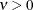in the Matérn type of semivariance structures. The special case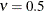is equivalent to the exponential model, whereas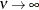gives the Gaussian model.

When you specify m different MATERN forms in the FORM= option, you must also provide m smoothness values in the SMOOTH option. If you must specify more than one smoothness value, the values are assigned sequentially to the MATERN nestings in the order the nestings are specified. If you specify more smoothness values than necessary, then values in excess are ignored.

STORESELECT(ssel-options)
SSEL(ssel-options)

specifies that information from an input item store be used for the prediction. You cannot combine the STORESELECT option with any of the FORM= or MDATA= options. The STORESELECT option has the following ssel-options:

TYPE=field-type

specifies whether to perform isotropic or anisotropic simulation. You can choose the field-type from one of the following:

ISO

specifies isotropic field for the simulation.

ANIGEO | GEO

specifies a field with geometric anisotropy for the simulation.

ANIZON(zonal-form1, …, zonal-formn)
ZON(zonal-form1, …, zonal-formn)

specifies a field with zonal anisotropy for the simulation. Each zonal-formi,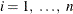, can be any of the following:

CUB | EXP | GAU | MAT | PEN | SHE | SPH

specify a form for the simulation.

Each zonal-formi,, is a structure in the purely zonal component of the correlation model in the direction angle of the minor anisotropy axis. For this reason, when you specify the TYPE=ANIZON suboption you must also specify the nonzonal component of the correlation model in the MODEL= suboption of the STORESELECT option. Assume the nonzonal component has k structures; these are common across all directions and each one has the same scale in all directions. In that sense, you use the TYPE=ANIZON suboption to specify only the n zonal anisotropy structures of an input store (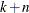)-structure nested model in the direction angle of the minor anisotropy axis.

Given this specification,must be up to the maximum number of nested model structures that is supported by the item store. See also the MODEL= suboption of the STORESELECT option.

In conclusion, you can use an input item store for prediction with zonal anisotropy if you know that every structure in the nonzonal model component has the same scale across all directions. When this condition does not apply for the item store models, specify the model parameters explicitly in the SIMULATE statement.

Computation of the MATERN covariance is numerically demanding. As a result, predictions that use Matérn covariance structures can be time-consuming.

If you omit the TYPE= option, the default behavior is TYPE=ISO when the input item store contains information for only one angle or for the omnidirectional case. If you specify an item store with information for more than one direction, then the default behavior is TYPE=ANIGEO.

When you specify TYPE=ISO to request isotropic analysis in the presence of an item store with information for multiple directions, you must specify the ANGLEID= suboption of the STORESELECT option with one argument. This argument specifies which of the direction angles information to use for the isotropic analysis.

When you indicate the presence of anisotropy with the TYPE=ANIGEO or TYPE=ANIZON suboptions of the STORESELECT option, the following conditions apply:

• You must specify the ANGLEID= suboption of the STORESELECT option to designate the major and minor anisotropy axes. See the ANGLEID= suboption of the STORESELECT option for details.

• For TYPE=ANIGEO, ensure that you have the same scale in all anisotropy directions.

• For TYPE=ANIZON, ensure that the nonzonal component scale is the same in all anisotropy directions.

If you import a nested model, these rules also apply to each one of the nested structures.

• Model ranges in the major anisotropy axis must be longer than ranges in the minor anisotropy axis.

• Any Matérn covariance structure must maintain its smoothness parameter value in all anisotropy directions.

ANGLEID=angleid1
ANGLEID=(angleid1, angleid2)

specifies which direction angles in the input item store be used for simulation. The angles are identified by the corresponding number in the AngleID column of the Store Models Information table, or by the AngleID parameter in the table title when you specify the INFO(DETAILS) option in the RESTORE statement.

If you request isotropic prediction in the TYPE= suboption of the STORESELECT option and the item store has omnidirectional contents or information about only one angle, then the ANGLEID= option is ignored. The simulation input comes from the omnidirectional information. In the case of a single angle, you still perform isotropic simulation and the model parameters are provided by the model in the single direction angle in the item store. However, if the item store contains information for more than one angle, then you must specify one angle ID in angleid1. The model information from the corresponding angle is then used in your isotropic simulation.

When you specify an anisotropic simulation in the TYPE= option of the STORESELECT option, you need to have information about two perpendicular direction angles. One of them is the major and the other is the minor anisotropy axis. You must always specify the major anisotropy axis angle ID in angleid1 and the minor anisotropy axis angle ID in angleid2. This means that the range parameters of the model forms in the angle designated by the angleid1 need to be larger than the corresponding ranges of the forms in the angle designated by the angleid2. Conveniently, if the item store has only two angles, then you only need to specify the ID angleid1 of the major anisotropy axis angle. If the item store has only one angle, then you cannot perform anisotropic simulation with input from the item store.

Note: You can perform geometric anisotropic analysis even if the item store does not contain information about a direction that is perpendicular to the one specified by angleid1. This is possible due to the geometry of the ellipse. In particular, when you specify the major axis with angleid1 and an angle ID for a second direction with a corresponding smaller range, then PROC SIM2D automatically computes the minor anisotropy axis range and the necessary range ratio parameter.

Anisotropic analysis is not possible when you specify instances of the same angle in the input item store. It is possible that PROC VARIOGRAM produces an item store where two or more directions can be the same if their corresponding correlation models were obtained for different angle tolerances or bandwidths in the VARIOGRAM procedure. Consequently, you cannot specify anisotropic simulation if the input store contains only two angles that are the same or if you specify angleid1 and angleid2 that correspond to equal angles.

MODEL=form
MODEL=(form1, …, formk)

specifies the theoretical semivariogram model selection to use for the simulation. Use any combination of one, two, or three forms to describe a model in the input item store because up to three nested structures are supported. Each formi,, can be any of the following:

CUB | EXP | GAU | MAT | PEN | SHE | SPH

specify the selection model.

Computation of the MATERN covariance is numerically demanding. As a result, simulations that use Matérn covariance structures can be time-consuming.

All fitted models that are stored in the input item store contain information about their component parameters and also about the nugget effect if any. The SIM2D procedure retrieves this information when you make a model selection in the MODEL= option, and you do not need to individually specify a nugget effect or any other parameter of the model.

By default, the model that is ranked first among the models for a given angle in the item store is used for the simulation task. If more than one model is available in the item store, then you can specify the MODEL= option to use a different model for the simulation.

In an anisotropic simulation, the default selection is the model that is ranked first in the direction angle of the major anisotropy axis. If you specify the TYPE=ANIGEO option, then a model that consists of identical structures needs to be present in the selected minor anisotropy axis angle in the item store. If you specify the TYPE=ANIZON option, then a model with the exact same first k structures must be present in the selected minor anisotropy axis angle, and it must feature at least one more structure as a zonal component. The zonal component is specified separately in the TYPE=ANIZON suboption of the STORESELECT option. Consequently, remember that in zonal anisotropy the MODEL= suboption designates only the nonzonal component of the correlation model in the minor anisotropy axis direction. In all, if there are k common structures and n structures in the purely zonal component, thenmust be up to the maximum number of nested model structures that is supported by the item store.

SVAR=store-var
SVAR=(store-varlist)

specifies one store-var item store variable or a list store-varlist of variables that are present in the item store. This option selects one or more item store variables whose correlation models you want to use in the current simulation task.

If you are performing a conditional simulation, then PROC SIM2D searches the input item store for the variable that is specified in the VAR= option of the SIMULATE statement. Then, the procedure selects the appropriate correlation model for the task. In this case, if you specify the SVAR= option, it is ignored. However, when you request an unconditional simulation and specify input from an item store, then you must also use SVAR= to specify a source for your correlation model.

In comparison to the other two ways of specifying a correlation model in PROC SIM2D, the STORESELECT option is quite different because you can avoid explicit specification of all parameter values of a model. When you specify the STORESELECT option, then the corresponding scale, range, nugget effect, and smoothness (if appropriate) parameter values are invoked as saved attributes of the model that you select from the item store.

In the case of anisotropy, you specify the angles indirectly with the ANGLEID= option of the STORESELECT option, and the ratios are computed implicitly by using the selected model ranges. Explore how to specify valid anisotropical models imported from an input item store with the two examples that follow.

In the first example, assume the input item store InStoreGeo contains exponential models in the angles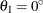,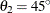, and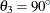. You know in advance that all models have the same scale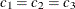across these directions and that the respective ranges are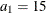,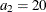, and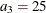in distance units. Hence, you have a case of geometric anisotropy where the major anisotropy axis is in the direction of angle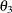and the minor anisotropy axis is in the direction of angle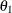. The following statements in PROC SIM2D use the information in the item store InStoreGeo to perform simulation under the assumption of geometric anisotropy:

proc sim2d data=...;
restore in=InStoreGeo;
simulate storeselect(model=exp type=anigeo angleid=(3,1));
run;


For the second example, assume a case of zonal anisotropy. Consider the input item store InStoreZon, which contains models in the two angles,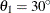and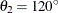. Specifically, inyou have an exponential-spherical model: the exponential structure has scale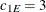and range; the spherical structure has scale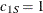and range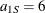. In direction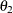you have an exponential model with scaleand range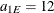. Hence, the zonal anisotropy major axis is in the direction of the lowest total variance, which is in angle; then, the minor axis is in the direction of angle. The following statements in PROC SIM2D use the information in the store InStoreZon to perform simulation under the assumption of zonal anisotropy:

proc sim2d data=...;
restore in=InStoreZon;
simulate storeselect(model=exp type=anizon(sph) angleid=(2,1));
run;